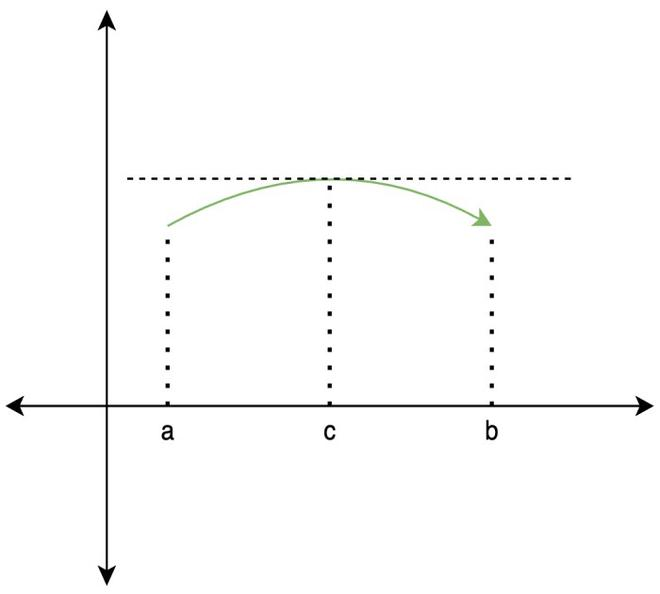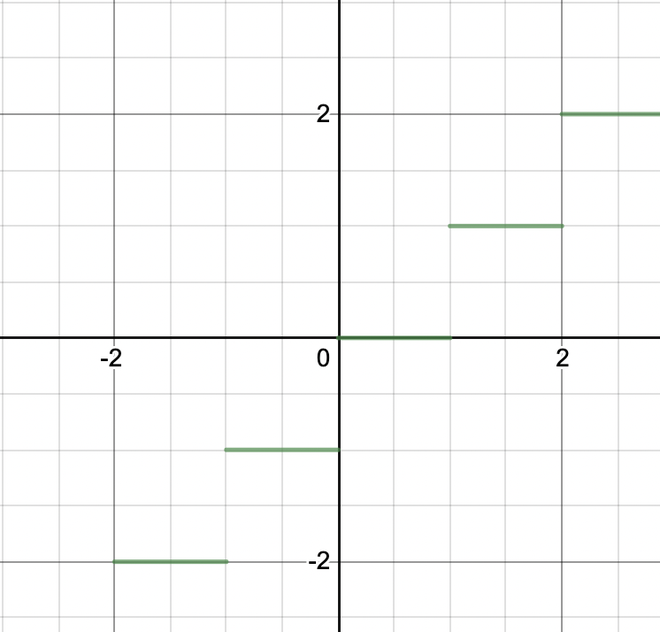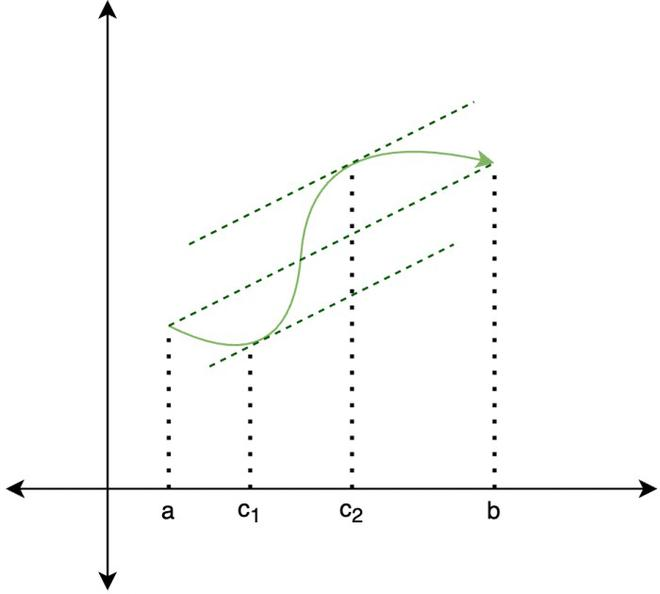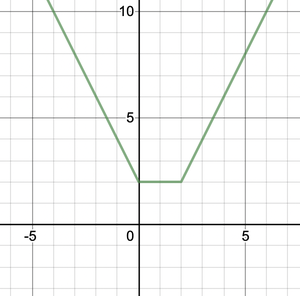# Rolle’s and Lagrange’s Mean Value Theorem

• Last Updated : 20 Sep, 2021

Mean Value Theorems (MVT) is one of the frequently occurring theorems in mathematics education literature. It is one of the most important tools used to prove a lot of other theorems in differential and integral calculus. It is sometimes taught with its special case, Rolle’s theorem. Rolle’s theorem is named after Michel Rolle (1652-1719), a French mathematician who established the now common symbol for the nth root and insisted that -a > -b, for positive a and b, a < b. The feat went against Descartes’ teaching and laid the groundwork for the introduction of the ubiquitous number line.

### Rolle’s Theorem

It states that if y = f(x) and an interval [a, b] is given and that it satisfies the following conditions:

1. f(x) is continuous in [a, b].
2. f(x) is differentiable in (a, b).
3. f(a) = f(b)

Then there exists at least one real number c ∈ (a, b) such that f'(c) = 0.Note: It is not necessary that the converse of this theorem is also true. That is we cannot say if at some point f'(c) = 0 then all the conditions of Rolle’s theorem are satisfied.

### Proof of Rolle’s Theorem

Let k = f(a) = f(b). We consider three cases:

1. f(x) = k for all x ∈ (a, b).
2. There exists an x ∈ (a, b) such that f(x) > k.
3. There exists an x ∈ (a, b) such that f(x) < k.

Case 1: If f(x) = k for all x ∈ (a, b), then f'(x) = 0 for all x ∈ (a, b).

Case 2: Since f is a continuous function over the closed, bounded interval[a, b], by the extreme value theorem, it has an absolute maximum. Also, since there is a point x ∈ (a, b) such that f(x) > k, the absolute maximum is greater than k. Therefore, the absolute maximum does not occur at either endpoint. As a result, the absolute maximum must occur at an interior point xc∈ (a, b). Because f has a maximum at an interior point c, and f is differentiable at c, by Fermat’s theorem, f'(c)=0.

Case 3: The case when there exists a point x ∈ (a, b) such that f(x) < k is analogous to case 2, with maximum replaced by minimum.

### Sample Problems

Question 1: Determine all the points ‘c’ which satisfy Rolle’s theorem for f(x) = x2 – 2x – 8 on [-1, 3].

Solution:

Before finding out the points, we need to make sure that all the conditions of rolle’s theorem are applied on this interval. This function is a polynomial, so it’s both differentiable and continuous on the interval. Now let’s evaluate the function at the ends of the interval. f(-1) = -5 and f(3) = -5. Function values are equal at both the ends. So, now all the conditions for rolle’s theorem are satisfied.

f'(x) = 2x – 2.

f'(x) = 2x – 2 = 0.

= x = 1

So, c = 1.

Question 2: Determine all the points ‘c’ which satisfy rolle’s theorem for f(x) = 2x- x2 – x3 on the interval [-2, 1].

Solution:

This function is also a polynomial, so it is both differential and continuous on the interval. Values at the end of the interval f(-2) = 0 = f(1).

So, Rolle’s theorem is applicable here.

f'(x) = 2 – 2x – 3x2

For finding the points like ‘c’

f'(x) = 0

⇒ 2 – 2x – 3x2 = 0

⇒ 3x2 + 2x -2 = 0

Applying the Shree Dharacharya quadratic formula for finding the values of c,So, here there are two values which satisfy rolle’s theorem.

Question 3: Examine if rolle’s theorem is applicable for the functions f(x) = [x] for x ∈ [5, 9].

Solution:

This function is a step function, it is not continuous. So, rolle’s theorem is not applicable in the following interval.f(x) = [x]

### Mean Value Theorem

It is one of the most important theorems in calculus. It states that if y = f(x) and an interval [a, b] is given and that it satisfies the following conditions:

• f(x) is continuous in [a, b].
• f(x) is differentiable in (a, b).

Then there exists at least one number c ∈ (a, b) such thatGeometrically speaking, the derivative at c denotes the slope of the tangent of at x = c for f(x). It says, there must exist a point in between that interval where the slope of the tangent is equal to the slope of the line joining points x = a and x = b.Note: There can be any number of c-points. The mean Value Theorem is also called First Mean Value Theorem.

Proof:

Let “f” satisfy the hypotheses of the MVT on the interval [a, b]. Define. This represents the secant line between a and b.

Case 1: g attains its maximum and minimum at a and b. Then “g” is constant. In this case we getat every point in [a, b]

Case 2: If the maximum or the minimum is attained at an interior point c, then g'(c)=0. Therefore,### Physical Interpretation of Mean Value Theorem

It is known that in Mean Value Theorem,is average change in function over [a, b], and f'(c) is the instantaneous change at ‘c’. It states Over the Interval instantaneous change is equal to the average change of the Function at some interior point.

### Application of Mean Value Theorem

Mean Value Theorem is known to be one of the most essential theorems in analysis and therefore, all its applications have major significance. Some of the applications are listed below:

• Leibniz’s Rule.
• L’ Hospital’s Rule.
• Strictly Increasing and Strictly decreasing functions.
• The symmetry of Second derivatives.
• The function is constant if f: (a, b)R is Differentiable and f'(x)=0 for all x\in(a, b).

### Sample Problems

Question 1: Determine all the number(s) “c” which satisfy the conclusion of Mean Value Theorem for h(z) = 4z3 – 8z2 + 7z – 2 on [2,5].

Solution:

Since the function is a polynomial, so it is continuous and differentiable both in this interval. Thus mean value theorem can be applied here.

h(2) = 12 and h(5) = 333 and h'(z) = 12z2 -16z + 7

Now plug this into the formula of mean value theorem and solve for c.Question 2: f(x) =. Find the interval over which mean value theorem can be applied.

Solution:

f(x) is not defined at x = 0. So, x = 0 is not in the domain if f(x).

Since MVT can only be defined at places where f(x) is differentiable and continuous. It can be defined (-∞, 0) and (0,∞).

Question 3: Determine all the number(s) “c” which satisfy the conclusion of Mean Value Theorem in the interval [-2,2] for f(x) =Solution:

The given function, f(x) = |x – 2| + |x| is not differentiable. Thus mean value theorem cannot be applied on this function in the interval [-2, 2].Graph of the given function.

Question 4: Determine all the number(s) “c” which satisfy the conclusion of Mean Value Theorem in the interval foron [-2,3].

Solution:

This function is a sum of both exponential and polynomial functions. Since both the functions are continuous and differentiable everywhere, the function f(t) is continuous and differentiable everywhere. Thus mean value theorem is applicable.

f(-2) = -16 + e(-3 .-2) = -16 + e6, f(3) = 24 + e-9 and f'(t) = 8 -3e-3t

Let’s plug it into the formula,This is the value of c.

My Personal Notes arrow_drop_up